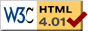### Journée-séminaire de combinatoire

#### (équipe CALIN du LIPN, université Paris-Nord, Villetaneuse)

Le 19 mars 2019 à 10h30 en B107, Christian Ikenmeyer nous parlera de : Inequalities between plethysm coefficients and Kronecker coefficients via geometric complexity theory

Résumé : Research on Kronecker coefficients and plethysms gained significant momentum when the topics were connected to geometric complexity theory, an approach towards computational complexity lower bounds via algebraic geometry and representation theory. Both types of coefficients appear independently in R. Stanley's list of "Positivity problems and conjectures in algebraic combinatorics". This talk is about a result that was obtained with geometric complexity theory as motivation, namely an inequality between rectangular Kronecker coefficients and plethysm coefficients. The proof interestingly uses insights from algebraic complexity theory. As far as we are aware, algebraic complexity theory has never been used before to prove an inequality between representation theoretic multiplicities. This is joint work with Greta Panova (Rectangular Kronecker coefficients and plethysms in geometric complexity theory, Adv Math 2017).

 Dernière modification : Monday 24 January 2022Contact pour cette page : Cyril.Banderier at lipn.univ-paris13.fr